Hits: 4

# Strongly Connected Components

#### In this tutorial, you will learn how strongly connected components are formed. Also, you will find working examples of kosararju’s algorithm in Python.

A strongly connected component is the portion of a directed graph in which there is a path from each vertex to another vertex. It is applicable only on a directed graph.

For example:

Let us take the graph below.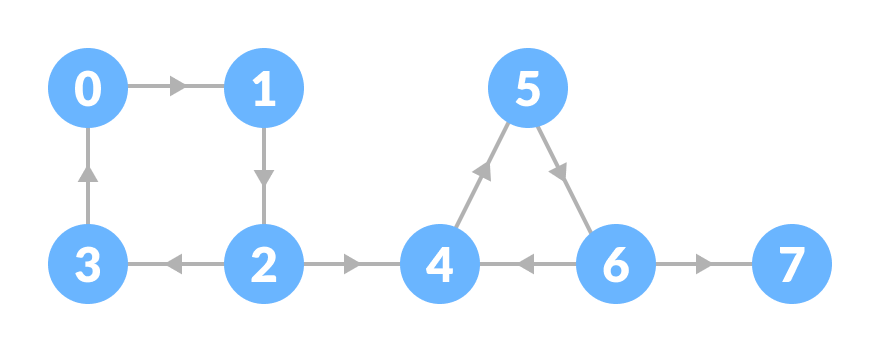Initial graph

The strongly connected components of the above graph are: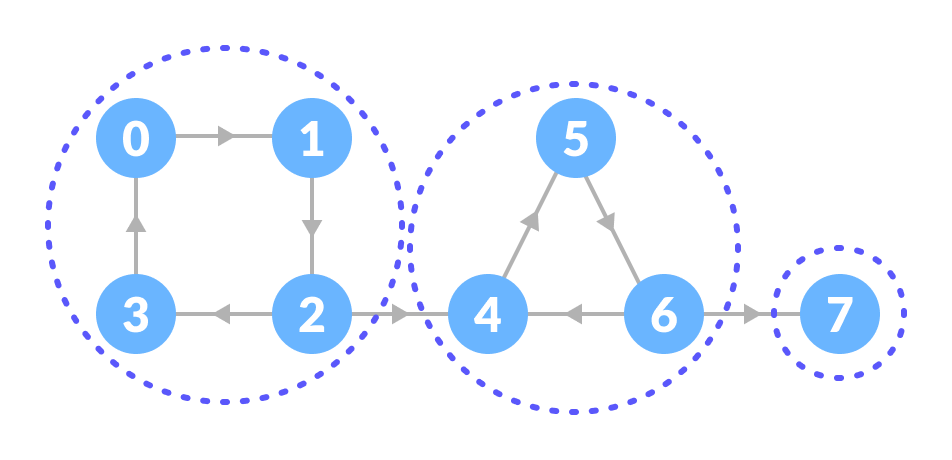Strongly connected components

You can observe that in the first strongly connected component, every vertex can reach the other vertex through the directed path.

These components can be found using Kosaraju’s Algorithm.

## Kosaraju’s Algorithm

Kosaraju’s Algorithm is based on the depth-first search algorithm implemented twice.

Three steps are involved.

1. Perform a depth first search on the whole graph.Let us start from vertex-0, visit all of its child vertices, and mark the visited vertices as done. If a vertex leads to an already visited vertex, then push this vertex to the stack.

For example: Starting from vertex-0, go to vertex-1, vertex-2, and then to vertex-3. Vertex-3 leads to already visited vertex-0, so push the source vertex (ie. vertex-3) into the stack.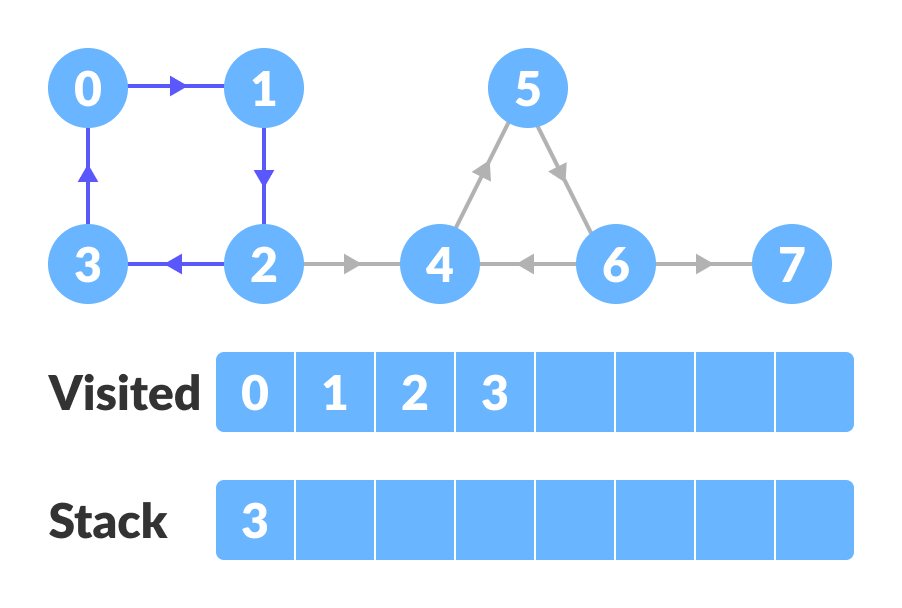DFS on the graph

Go to the previous vertex (vertex-2) and visit its child vertices i.e. vertex-4, vertex-5, vertex-6 and vertex-7 sequentially. Since there is nowhere to go from vertex-7, push it into the stack.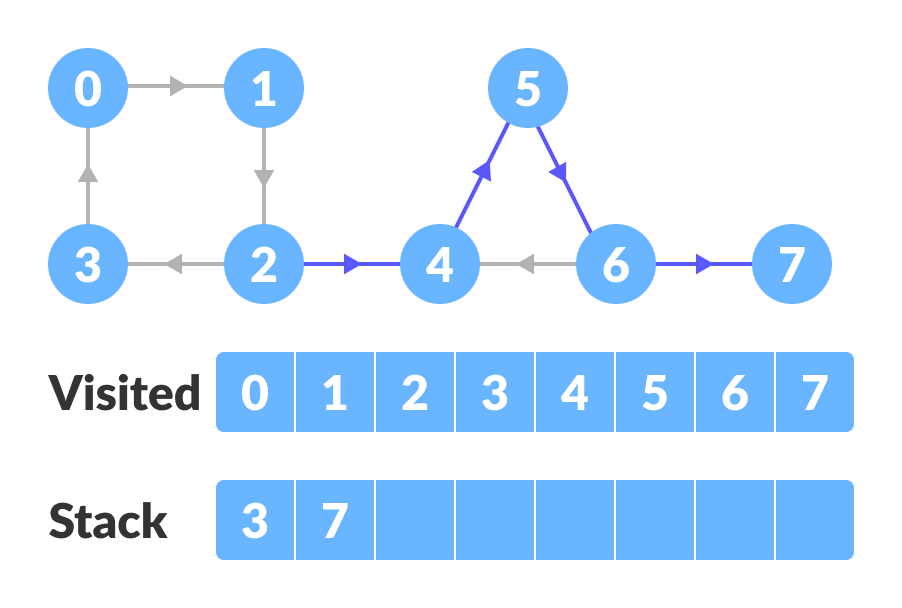DFS on the graph

Go to the previous vertex (vertex-6) and visit its child vertices. But, all of its child vertices are visited, so push it into the stack.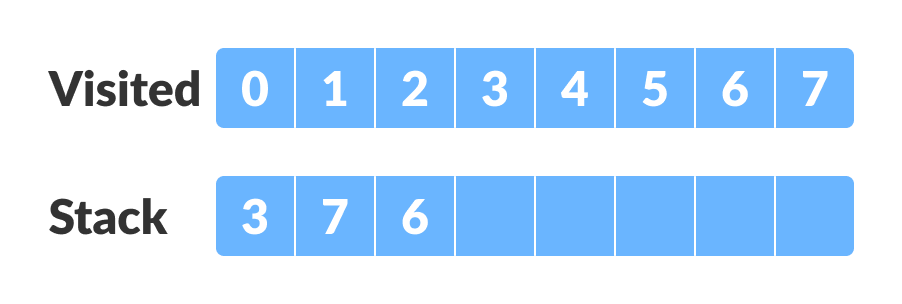Stacking

Similarly, a final stack is created.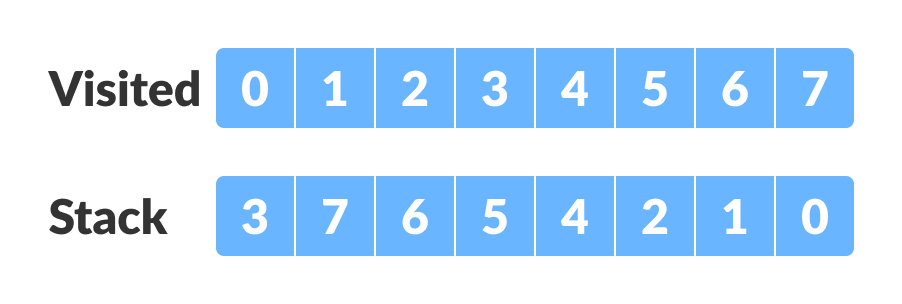Final Stack
2. Reverse the original graph.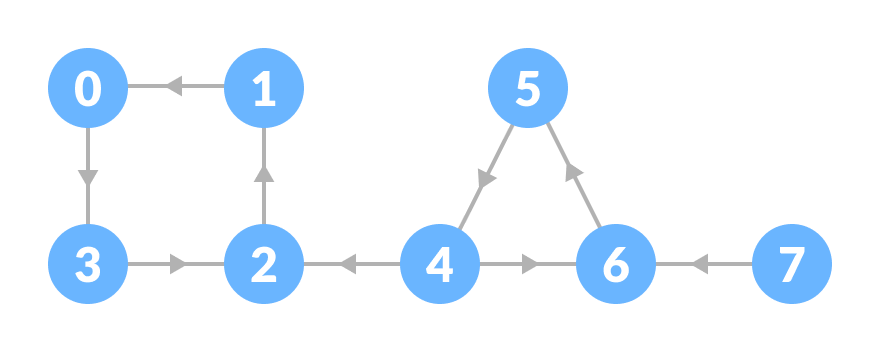DFS on reversed graph
3. Perform depth-first search on the reversed graph.Start from the top vertex of the stack. Traverse through all of its child vertices. Once the already visited vertex is reached, one strongly connected component is formed.

For example: Pop vertex-0 from the stack. Starting from vertex-0, traverse through its child vertices (vertex-0, vertex-1, vertex-2, vertex-3 in sequence) and mark them as visited. The child of vertex-3 is already visited, so these visited vertices form one strongly connected component.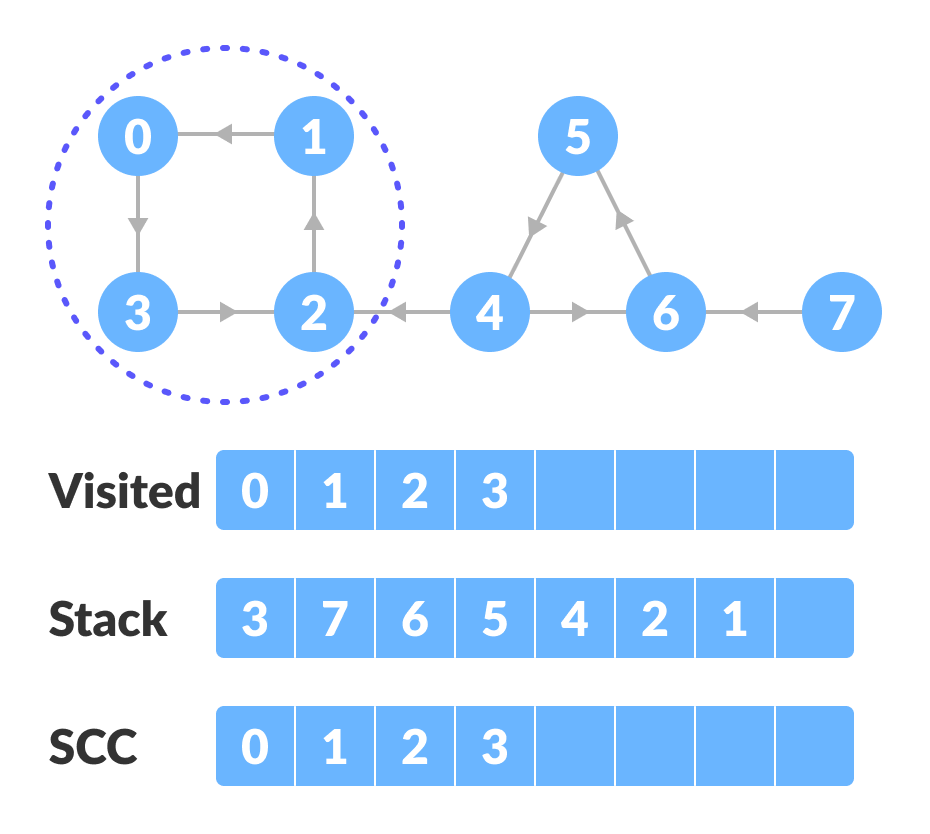Start from the top and traverse through all the vertices

Go to the stack and pop the top vertex if already visited. Otherwise, choose the top vertex from the stack and traverse through its child vertices as presented above.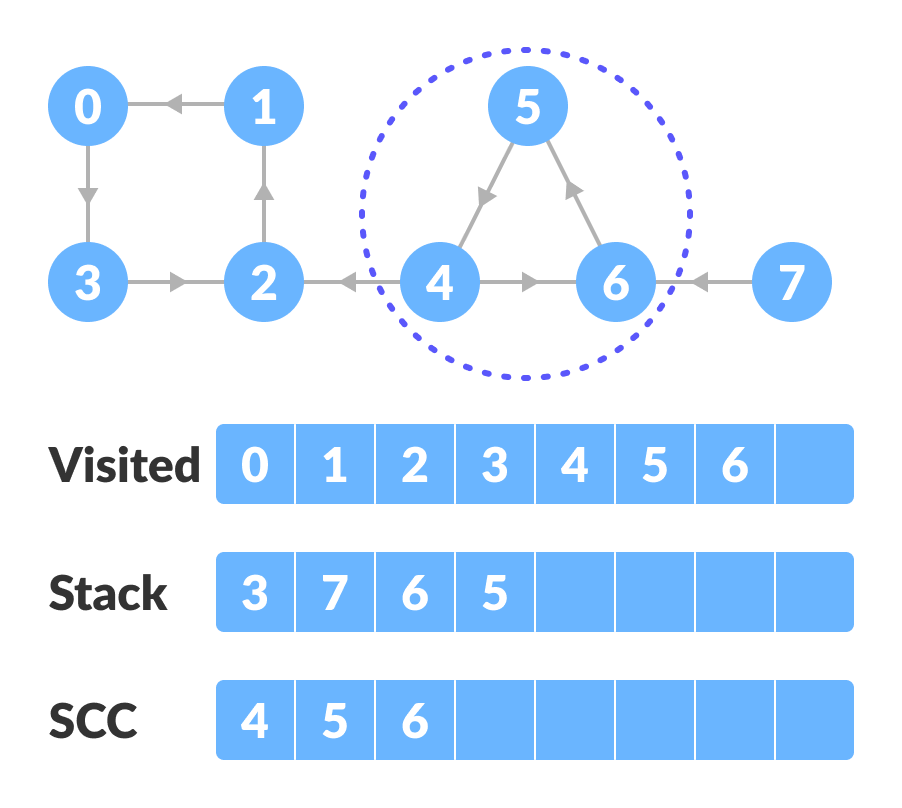Pop the top vertex if already visited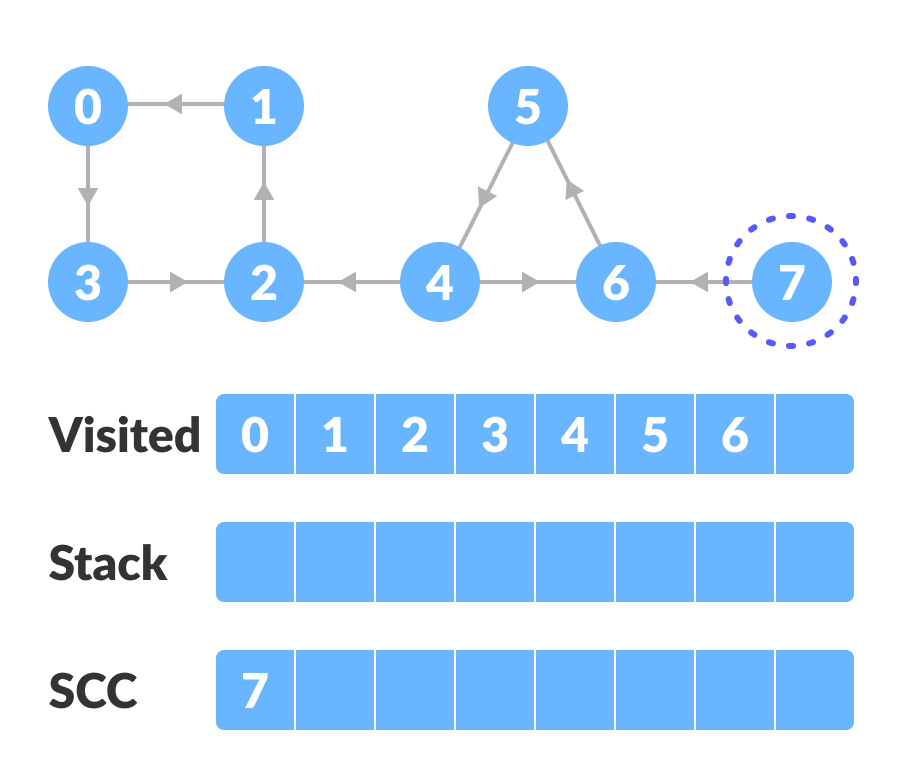Strongly connected component
4. Thus, the strongly connected components are:All strongly connected components

## Python Examples

``````/* Kosaraju's algorithm to find strongly connected components in Python */

from collections import defaultdict

class Graph:

def __init__(self, vertex):
self.V = vertex
self.graph = defaultdict(list)

/* Add edge into the graph */
def add_edge(self, s, d):
self.graph[s].append(d)

/* dfs */
def dfs(self, d, visited_vertex):
visited_vertex[d] = True
print(d, end='')
for i in self.graph[d]:
if not visited_vertex[i]:
self.dfs(i, visited_vertex)

def fill_order(self, d, visited_vertex, stack):
visited_vertex[d] = True
for i in self.graph[d]:
if not visited_vertex[i]:
self.fill_order(i, visited_vertex, stack)
stack = stack.append(d)

/* transpose the matrix */
def transpose(self):
g = Graph(self.V)

for i in self.graph:
for j in self.graph[i]:
g.add_edge(j, i)
return g

/* Print stongly connected components */
def print_scc(self):
stack = []
visited_vertex = [False] * (self.V)

for i in range(self.V):
if not visited_vertex[i]:
self.fill_order(i, visited_vertex, stack)

gr = self.transpose()

visited_vertex = [False] * (self.V)

while stack:
i = stack.pop()
if not visited_vertex[i]:
gr.dfs(i, visited_vertex)
print("")

g = Graph(8)
g.add_edge(0, 1)
g.add_edge(1, 2)
g.add_edge(2, 3)
g.add_edge(2, 4)
g.add_edge(3, 0)
g.add_edge(4, 5)
g.add_edge(5, 6)
g.add_edge(6, 4)
g.add_edge(6, 7)

print("Strongly Connected Components:")
g.print_scc()``````

## Kosaraju’s Algorithm Complexity

Kosaraju’s algorithm runs in linear time i.e. `O(V+E)`.

## Strongly Connected Components Applications

• Vehicle routing applications
• Maps
• Model-checking in formal verification

# Special 95% discount

## 2000+ Applied Machine Learning & Data Science Recipes

### Portfolio Projects for Aspiring Data Scientists: Tabular Text & Image Data Analytics as well as Time Series Forecasting in Python & R## Two Machine Learning Fields

There are two sides to machine learning:

• Practical Machine Learning:This is about querying databases, cleaning data, writing scripts to transform data and gluing algorithm and libraries together and writing custom code to squeeze reliable answers from data to satisfy difficult and ill defined questions. It’s the mess of reality.
• Theoretical Machine Learning: This is about math and abstraction and idealized scenarios and limits and beauty and informing what is possible. It is a whole lot neater and cleaner and removed from the mess of reality.

Data Science Resources: Data Science Recipes and Applied Machine Learning Recipes

Introduction to Applied Machine Learning & Data Science for Beginners, Business Analysts, Students, Researchers and Freelancers with Python & R Codes @ Western Australian Center for Applied Machine Learning & Data Science (WACAMLDS) !!!

Latest end-to-end Learn by Coding Recipes in Project-Based Learning:

Applied Statistics with R for Beginners and Business Professionals

Data Science and Machine Learning Projects in Python: Tabular Data Analytics

Data Science and Machine Learning Projects in R: Tabular Data Analytics

Python Machine Learning & Data Science Recipes: Learn by Coding

R Machine Learning & Data Science Recipes: Learn by Coding

Comparing Different Machine Learning Algorithms in Python for Classification (FREE)

`Disclaimer: The information and code presented within this recipe/tutorial is only for educational and coaching purposes for beginners and developers. Anyone can practice and apply the recipe/tutorial presented here, but the reader is taking full responsibility for his/her actions. The author (content curator) of this recipe (code / program) has made every effort to ensure the accuracy of the information was correct at time of publication. The author (content curator) does not assume and hereby disclaims any liability to any party for any loss, damage, or disruption caused by errors or omissions, whether such errors or omissions result from accident, negligence, or any other cause. The information presented here could also be found in public knowledge domains.  `